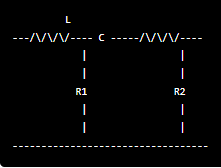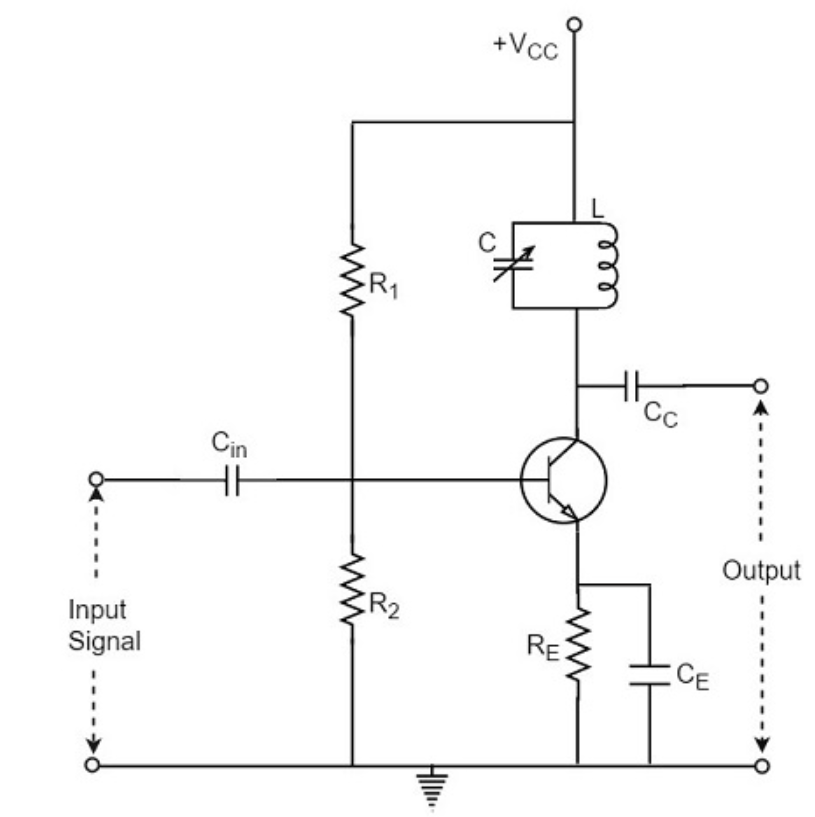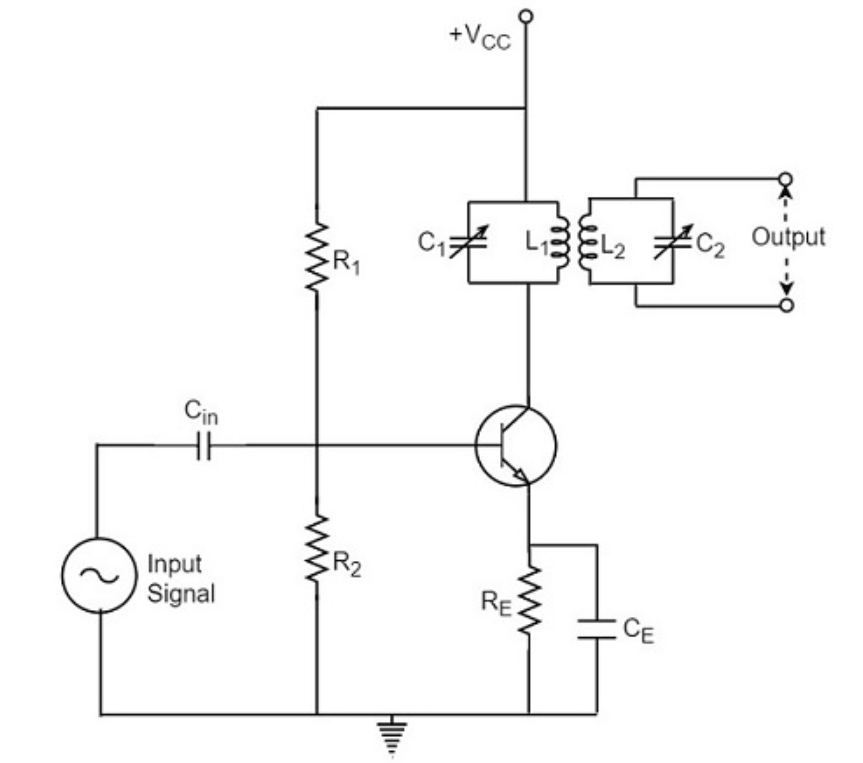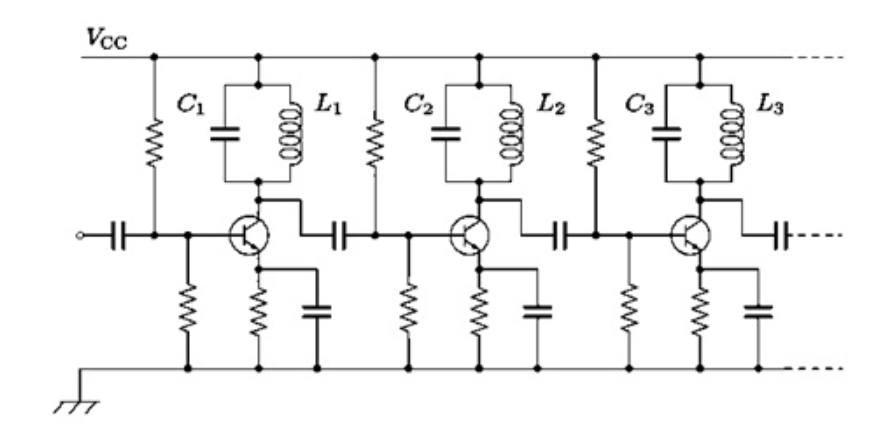Select Page

# Tuned Amplifiers

Analog Circuits: Tuned Amplifiers

Contents

Describe Tuned Amplifiers 3

Describe the concept of Resonance in Tuned Amplifiers 4

Derive an expression for Series Resonant Frequency 5

Determine the relationship between Q-factor, Bandwidth, and Resonant Frequency 6

Derive an expression for Parallel Resonant Frequency 7

Determine the relationship between Q-factor, Bandwidth, and Resonant Frequency 9

Describe Single Tuned Amplifier with circuit diagram 9

Determine Voltage gain & Frequency response of Single Tuned Amplifiers 10

Describe Double Tuned Amplifier with circuit diagram 13

Determine Voltage gain and Frequency response of Double Tuned Amplifier 14

Describe Staggered-tuned Amplifier with the help of Circuit diagram 17

Determine Voltage-gain & Frequency response of Staggered-tuned Amplifier 18

Describe the term Neutralisation in Tuned Amplifiers 20

# Describe Tuned Amplifiers

Tuned amplifiers are a type of electronic amplifier that utilize a resonant circuit, also known as a tank circuit, to amplify a specific frequency range. The resonant circuit consists of an inductor and a capacitor, which resonate at a specific frequency called the resonant frequency. Tuned amplifiers are used in radio frequency (RF) communication systems, such as radios and televisions, to amplify the signal in a particular frequency band.

There are two main types of tuned amplifiers: parallel-tuned and series-tuned. In a parallel-tuned amplifier, the resonant circuit is connected in parallel with the amplifier’s input and output, while in a series-tuned amplifier, the resonant circuit is connected in series with the amplifier’s input and output. The choice of configuration depends on the application and the desired performance characteristics.

The performance of a tuned amplifier is characterized by its Q-factor, which is a measure of the selectivity of the resonant circuit. A high Q-factor indicates that the amplifier is highly selective and amplifies a narrow frequency range, while a low Q-factor indicates that the amplifier is less selective and amplifies a wider frequency range. The Q-factor of a tuned amplifier can be adjusted by varying the values of the inductor and capacitor in the resonant circuit.

Tuned amplifiers are widely used in RF communication systems due to their ability to provide high selectivity and gain in a specific frequency range. However, they are also susceptible to frequency drift and require careful tuning to maintain their performance.

Applications of Tuned Amplifiers:

• In radio receivers for selective amplification of a particular frequency band.
• In audio systems to amplify a specific frequency range.
• In electronic oscillators to provide feedback for sustaining oscillations.
• In signal processing circuits for filtering and frequency discrimination.

• Selective amplification of a particular frequency band without affecting other frequencies.
• High gain at the center frequency of the tuned circuit.
• Low noise and distortion.

• Narrow bandwidth, which limits the range of frequencies that can be amplified.
• Sensitivity to temperature changes, component variations, and external interference, which can affect the resonant frequency of the tuned circuit.
• Limited dynamic range, which can cause distortion at high input levels.

# Describe the concept of Resonance in Tuned Amplifiers

Resonance is a phenomenon in which an electrical circuit or mechanical system vibrates at its natural frequency in response to an external stimulus. In the case of tuned amplifiers, resonance occurs when the reactive elements in the tuned circuit (inductor and capacitor) are adjusted to a specific frequency, known as the resonant frequency, at which the circuit exhibits maximum impedance.

When an AC signal is applied to the input of a tuned amplifier, the voltage across the tuned circuit depends on the frequency of the input signal. At the resonant frequency, the voltage across the tuned circuit is maximum, and the impedance of the circuit is minimum. This allows the maximum amount of signal power to be transferred to the next stage of the amplifier, resulting in maximum amplification of the desired frequency.

The bandwidth of a tuned amplifier is defined as the range of frequencies around the resonant frequency at which the output power is at least half of the maximum power. The Q factor, or quality factor, of the tuned circuit is a measure of its selectivity and is defined as the ratio of the resonant frequency to the bandwidth. Higher Q circuits have a narrower bandwidth and better selectivity.

Overall, the concept of resonance is critical to the functioning of tuned amplifiers, as it allows for selective amplification of specific frequencies while rejecting unwanted frequencies.

# Derive an expression for Series Resonant Frequency

To derive an expression for the series resonant frequency, let’s consider an RLC series circuit consisting of a resistor (R), inductor (L), and capacitor (C) connected in series. The resonant frequency is the frequency at which the circuit exhibits maximum impedance or minimum current.

The impedance of the RLC series circuit is given by the following equation:

Z = R + j(XL – XC)

Where:

Z is the impedance of the circuit,

R is the resistance,

XL is the inductive reactance,

XC is the capacitive reactance, and

j is the imaginary unit (√(-1)).

At the resonant frequency, the inductive reactance (XL) and the capacitive reactance (XC) are equal in magnitude but opposite in sign. Mathematically, XL = -XC.

Substituting this condition into the impedance equation, we have:

Z = R + j(XL – XC)

Z = R + j(0)

Z = R

At the resonant frequency, the impedance of the circuit is purely resistive, and there is no reactive component. Therefore, the series resonant frequency can be determined by equating the inductive and capacitive reactances and solving for the frequency.

XL = XC

ωL = 1/ωC

Where:

ω is the angular frequency in radians per second,

L is the inductance in Henry (H), and

C is the capacitance in Farad (F).

Simplifying the equation, we have:

ω = 1/√(LC)

The series resonant frequency (fr) is given by:

fr = ω / (2π)

Substituting the expression for ω, we get:

fr = (1/√(LC)) / (2π)

Simplifying further, we can express the series resonant frequency as:

fr = 1 / (2π√(LC))

This is the expression for the series resonant frequency of an RLC series circuit. It represents the frequency at which the inductive and capacitive reactances cancel each other out, resulting in maximum impedance or minimum current in the circuit.

# Determine the relationship between Q-factor, Bandwidth, and Resonant Frequency

The relationship between Q-factor, bandwidth, and resonant frequency in a tuned circuit can be expressed mathematically as follows:

Q = fresonant / bandwidth

where Q is the quality factor, fresonant is the resonant frequency, and bandwidth is the width of the frequency range over which the circuit resonates.

Alternatively, we can rearrange the equation to solve for one of the other variables:

fresonant = Q x bandwidth

or

bandwidth = fresonant / Q

This equation tells us that the higher the Q-factor of the tuned circuit, the narrower the bandwidth of the circuit. This means that the circuit will resonate at a very specific frequency, and will not respond as strongly to frequencies outside of this narrow range.
Conversely, if we want a wider bandwidth, we can either decrease the Q-factor or increase the resonant frequency. However, doing so will also decrease the selectivity of the circuit, which means it will not be able to filter out unwanted frequencies as effectively.

# Derive an expression for Parallel Resonant Frequency

To derive an expression for the parallel resonant frequency, let’s consider the following parallel RLC circuit:Where:

• R1 and R2 are the resistors in parallel.
• L is the inductor.
• C is the capacitor.

The total impedance of the circuit is:
markdown

Z = R1 || R2 + jωL + 1/(jωC)

Where “||” represents the parallel combination.

We want to find the frequency at which the circuit is resonant, i.e., the frequency at which the impedance is purely resistive. This happens when the imaginary part of the impedance is zero, so:

markdown

jωL + 1/(jωC) = 0

Multiplying both sides by jωC:

markdown

jωL jωC + 1 = 0

Solving for ω:

markdown

jωL jωC = -1 ω2 = 1/(LC)

Therefore, the parallel resonant frequency is:

markdown

fres = 1 / (2π √(LC))

where fres is the frequency of resonance, L is the inductance, and C is the capacitance.

# Determine the relationship between Q-factor, Bandwidth, and Resonant Frequency

The relationship between Q-factor, bandwidth, and resonant frequency for a parallel resonant circuit is given as:

Q-factor = Resonant frequency / Bandwidth

Bandwidth = Resonant frequency / Q-factor

Resonant frequency = 1 / [2 * pi * sqrt(L * C)]

where L is the inductance of the circuit, C is the capacitance of the circuit.

From these equations, we can see that the Q-factor and resonant frequency are inversely proportional to the bandwidth. This means that a circuit with a high Q-factor will have a narrow bandwidth and a high resonant frequency, while a circuit with a low Q-factor will have a wider bandwidth and a lower resonant frequency.

# Describe Single Tuned Amplifier with circuit diagram

A single tuned amplifier is a type of tuned amplifier that consists of an LC circuit tuned to the desired frequency of the input signal, and an amplifier stage. The circuit diagram of a single tuned amplifier is shown below:The input signal is applied to the inductor L, which is in series with the capacitor C. The LC circuit acts as a filter that passes the desired frequency while rejecting other frequencies. The resistor R is the load of the amplifier and the output signal is taken across it. The amplifier stage is placed between the LC circuit and the load resistor R.

The performance of a single tuned amplifier can be improved by using negative feedback. A portion of the output signal is fed back to the input of the amplifier with opposite polarity to the input signal. This reduces distortion and improves the amplifier’s frequency response.

# Determine Voltage gain & Frequency response of Single Tuned Amplifiers

To determine the voltage gain and frequency response of a single tuned amplifier, we need to analyze the circuit and derive the relevant equations.

The circuit diagram of a single tuned amplifier is as follows:Where:

The output can be obtained from the coupling capacitor CC as shown above or from a secondary winding placed at L.

A single-tuned amplifier is a type of tuned amplifier circuit that uses a single-tuned circuit for the input and output stages. The input stage of the amplifier is tuned to the frequency of the input signal, while the output stage is tuned to the frequency of the output signal. The tuning circuit consists of a resonant circuit, typically an LC circuit.

The voltage gain and frequency response of a single-tuned amplifier depend on the values of the components in the tuning circuit, as well as the load resistance and the characteristics of the amplifier.

To determine the voltage gain of a single-tuned amplifier, we can use the following formula:

Av = -RL / R1 * sqrt(L / C) * (1 / (R1 + R2 + RL + Rf))

where Av is the voltage gain, RL is the load resistance, R1 is the resistance of the input stage, R2 is the resistance of the output stage, Rf is the feedback resistance, L is the inductance of the tuning circuit, and C is the capacitance of the tuning circuit.

To determine the frequency response of a single-tuned amplifier, we can plot the gain versus frequency curve using the following formula:

Av = -RL / R1 * sqrt(L / C) * (1 / sqrt((1 – (f / fc)^2)^2 + (BW / fc)^2))

where f is the frequency, fc is the resonant frequency of the tuning circuit, and BW is the bandwidth of the amplifier.

The plot of the gain versus frequency curve typically shows a peak at the resonant frequency, which is the frequency at which the gain is maximum. The bandwidth of the amplifier is the range of frequencies over which the gain is greater than or equal to 70.7% of the maximum gain.

In practical circuits, the frequency response of a single-tuned amplifier may be affected by factors such as component tolerances, parasitic capacitances and inductances, and the non-ideal characteristics of the amplifier. Therefore, it is important to carefully design and test the circuit to ensure that it meets the desired performance specifications.

Single tuned amplifiers are commonly used in various applications, such as radio receivers, communication systems, and audio amplifiers. They have some advantages and disadvantages, which include:

• Single tuned amplifiers are relatively simple in design, making them cost-effective and easy to implement.
• They can provide a high level of selectivity and sensitivity, allowing for efficient filtering of specific frequencies.
• Single tuned amplifiers have a high Q-factor, which provides a narrow bandwidth and improves their frequency response.

• Single tuned amplifiers are sensitive to component tolerances and can be affected by temperature changes, making them less reliable.
• They have a limited frequency range due to their narrow bandwidth, which can be a drawback in some applications.
• Single tuned amplifiers can be susceptible to noise and interference, affecting their overall performance.

Applications:

• Single tuned amplifiers are commonly used in radio frequency (RF) systems, such as radio receivers and transmitters.
• They are used in audio amplifiers, especially in high-fidelity systems, to provide efficient filtering and amplification of specific frequencies.
• Single tuned amplifiers are used in communication systems, such as telecommunication and satellite systems, to filter and amplify specific signals.

# Describe Double Tuned Amplifier with circuit diagram

A double tuned amplifier is a type of tuned amplifier that uses two tuned circuits to achieve a higher level of selectivity and gain than a single tuned amplifier. The circuit diagram of a double tuned amplifier consists of two tuned circuits, each with a capacitor and an inductor in series. The two tuned circuits are connected in parallel, with the input signal being applied across one tuned circuit, and the output signal being taken from the other tuned circuit.

The double tuned amplifier is commonly used in radio frequency (RF) applications such as high frequency amplifiers, radio transmitters, and receivers. It provides a high degree of selectivity, allowing only a narrow range of frequencies to pass through the circuit, while attenuating unwanted frequencies. The double tuned amplifier also provides a high level of gain, making it suitable for use in high frequency circuits where low signal levels are present.

One advantage of the double tuned amplifier is that it provides a higher level of selectivity than a single tuned amplifier. This means that it is able to filter out unwanted frequencies more effectively, leading to a clearer output signal. However, this increased selectivity can also be a disadvantage in some applications, where a wider range of frequencies needs to be passed through the circuit.

Another advantage of the double tuned amplifier is its high gain, which makes it suitable for use in low signal level applications. However, this high gain can also lead to instability and oscillation in the circuit, particularly at high frequencies.
In summary, the double tuned amplifier is a type of tuned amplifier that uses two tuned circuits to provide a high level of selectivity and gain. It is commonly used in high frequency RF applications, and provides advantages such as high selectivity and gain, but can also have disadvantages such as instability and oscillation at high frequencies.

# Determine Voltage gain and Frequency response of Double Tuned Amplifier

In a double tuned amplifier, two resonant circuits are used to improve the selectivity of the amplifier. The circuit diagram of a double tuned amplifier is shown below:The resonant circuits L1C1C3 and L2C2C5 are tuned to the desired frequency. C4 provides bypassing to ground for the signal at the mid-frequency between the two resonant frequencies, thus preventing loading of the circuit. The voltage gain of the amplifier is given by:

Av = -R2 / R1

A double-tuned amplifier is a type of tuned amplifier circuit that uses two tuned circuits for the input and output stages. The input and output circuits are each tuned to a specific frequency, and the two tuning circuits are coupled together to provide additional selectivity and improved frequency response.

To determine the voltage gain of a double-tuned amplifier, we can use the following formula:

Av = -Q1 * Q2 * RL / R1 * sqrt(L1 / C1 * L2 / C2) * (1 / (R1 + R2 + RL + Rf))

where Av is the voltage gain, Q1 and Q2 are the quality factors of the input and output tuning circuits, RL is the load resistance, R1 is the resistance of the input stage, R2 is the resistance of the output stage, Rf is the feedback resistance, L1 and L2 are the inductances of the input and output tuning circuits, and C1 and C2 are the capacitances of the input and output tuning circuits.

To determine the frequency response of a double-tuned amplifier, we can plot the gain versus frequency curve using the following formula:

Av = -Q1 * Q2 * RL / R1 * sqrt(L1 / C1 * L2 / C2) * (1 / sqrt((1 – (f / f0)^2)^2 + (1 / Q1 * 1 / Q2 * (f / f0 – f0 / f)^2)))

where f is the frequency, f0 is the resonant frequency of the tuning circuits, and Q1 and Q2 are the quality factors of the input and output tuning circuits.

The plot of the gain versus frequency curve typically shows two peaks at the resonant frequencies of the input and output tuning circuits, which are the frequencies at which the gain is maximum. The bandwidth of the amplifier is determined by the Q factors of the tuning circuits and the coupling between the circuits.

In practical circuits, the frequency response of a double-tuned amplifier may be affected by factors such as component tolerances, parasitic capacitances and inductances, and the non-ideal characteristics of the amplifier. Therefore, it is important to carefully design and test the circuit to ensure that it meets the desired performance specifications.

Double-tuned amplifiers are used in radio frequency applications and have the advantage of high selectivity, as well as good stability and gain characteristics. However, they also have a narrow bandwidth, making them unsuitable for wider frequency ranges. Some of the advantages, disadvantages, and applications of double-tuned amplifiers are:

• High selectivity: Double-tuned amplifiers have a narrow bandwidth, which allows them to selectively amplify signals at a specific frequency.
• Good stability: The use of two tuned circuits in a double-tuned amplifier provides good stability against variations in component values and temperature changes.
• Good gain characteristics: Double-tuned amplifiers can provide high voltage gain at the resonant frequency.

• Narrow bandwidth: The narrow bandwidth of double-tuned amplifiers makes them unsuitable for wider frequency ranges.
• Complex design: The use of two tuned circuits in a double-tuned amplifier makes the design more complex than that of a single-tuned amplifier.

Applications:

• Radio frequency (RF) amplification: Double-tuned amplifiers are commonly used in RF amplifiers for their high selectivity and good gain characteristics.
• Filters: Double-tuned amplifiers can also be used as filters in RF applications, due to their narrow bandwidth and high selectivity.
• Oscillators: Double-tuned amplifiers can be used as oscillators in some RF applications, due to their ability to provide high voltage gain at the resonant frequency.

# Describe Staggered-tuned Amplifier with the help of Circuit diagram

The Staggered-tuned Amplifier is a type of RF amplifier that uses multiple tuned circuits to amplify a narrow band of frequencies with high gain and selectivity. The circuit diagram of a typical staggered-tuned amplifier is shown below:The circuit consists of four tuned circuits (L1C2, L2C4, L3C6, and L4C8) connected in series between the input and output terminals of the amplifier. Each tuned circuit consists of an inductor (L1, L2, L3, and L4) and a capacitor (C2, C4, C6, and C8) that are resonant at the desired frequency. The values of the inductors and capacitors are staggered such that adjacent circuits are resonant at slightly different frequencies.

The input signal is applied to the first tuned circuit (L1C2), and the output signal is taken from the last tuned circuit (L4C8). The amplifier is biased by a DC voltage (+Vcc) applied through resistor R1, and the bias is stabilized by capacitor C1. The amplifier stage is composed of a common-emitter amplifier with a bypass capacitor C3 that provides AC coupling. Resistors R2, R3, and R4 are used to provide voltage dividers and DC biasing for the various amplifier stages.

The staggered-tuned amplifier provides high gain and selectivity because each tuned circuit provides both amplification and filtering of the input signal. The staggered tuning ensures that adjacent circuits do not overlap too much, providing better selectivity and rejection of unwanted frequencies.

# Determine Voltage-gain & Frequency response of Staggered-tuned Amplifier

To determine the voltage gain and frequency response of a staggered-tuned amplifier, we need to consider the circuit configuration and the characteristics of its components.

A staggered-tuned amplifier is a multi-stage amplifier that consists of several tuned amplifier stages connected in cascade. Each stage is designed to have a different center frequency, and the input signal is staggered across these frequencies to achieve a wide overall bandwidth. Here, we’ll focus on analyzing the voltage gain and frequency response of a single stage in the staggered-tuned amplifier.

The voltage gain (Av) of a single stage can be determined by the following formula:

Av = -gm * RL

Where:

• gm is the transconductance of the active device in the stage (such as a transistor or vacuum tube),
• RL is the load resistance connected to the output of the stage.

The frequency response of the stage can be obtained by considering the input and output impedance characteristics, as well as the tuned circuit components (such as inductors and capacitors) used in the stage.

Typically, each stage in a staggered-tuned amplifier is designed as a bandpass filter with a certain center frequency and bandwidth. The frequency response of the stage will exhibit a peak at the center frequency and gradually roll-off as the frequency moves away from the center.

To analyze the frequency response, we consider the impedance characteristics of the tuned circuit in the stage. This involves calculating the input and output impedance at different frequencies and evaluating the overall response.

It’s important to note that the voltage gain and frequency response of a staggered-tuned amplifier depend on the specific design parameters, component values, and configuration of the amplifier stages. Therefore, a detailed analysis requires knowledge of the specific circuit implementation and component characteristics.

In practice, the voltage gain and frequency response of a staggered-tuned amplifier are determined through simulations, measurements, and optimization techniques to achieve the desired performance and meet the design specifications.

Staggered-tuned amplifiers are used to provide wide bandwidth, high gain, and high selectivity. Here are some advantages and disadvantages of staggered-tuned amplifiers:

1. Wide bandwidth: Staggered-tuned amplifiers have a wider bandwidth compared to single and double-tuned amplifiers.
2. High gain: They provide high voltage gain due to the presence of multiple tuned circuits.
3. High selectivity: Staggered-tuned amplifiers have a high degree of selectivity due to the presence of multiple tuned circuits.
4. Low noise: These amplifiers provide a low noise figure due to the use of tuned circuits that attenuate signals outside the passband.

1. Complex design: Staggered-tuned amplifiers require multiple tuned circuits, which makes the design more complex.
2. Sensitivity to component tolerances: These amplifiers are sensitive to component tolerances, which can affect their performance.
3. High cost: Due to the complex design and multiple tuned circuits, staggered-tuned amplifiers can be expensive to manufacture.

Applications: